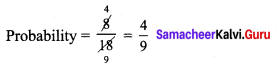## Tamilnadu Samacheer Kalvi 9th Maths Solutions Chapter 9 Probability Additional Questions

EXERCISE 9.1

Question 1.
An unbiased die is thrown. What is the probability of getting
(i) an even number or a multiple of 3.
(ii) a number between 3 and 6.
Solution:
(i) Probability of getting an even number $$\frac{3}{6}=\frac{1}{2}$$
Probability of getting a multiple of 3 = $$\frac{2}{6}$$
Probability of getting an even multiple of 3 = $$\frac{1}{6}$$
Probability of getting an even number or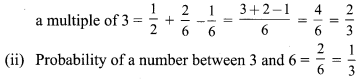Question 2.
Two unbiased coins are tossed simultaneously find the probability of getting
Solution:
S = {HH, HT, TH, TT}
(i) probability of two heads = $$\frac{1}{4}$$
(ii) probability of one head = $$\frac{1}{2}$$
(iii) probability of at least one head = $$\frac{3}{4}$$
(iv) probability of at most one head = $$\frac{3}{4}$$

Question 3.
Find the probability that a leap year selected at random will contain 53 Sundays.
Solution:
S = {Sunday Monday, Monday Tuesday, Tuesday Wednesday, Wednesday Thursday, Thursday Friday, Friday Saturday, Saturday Sunday}
n (S) = 7; n (A) = 2; P(A) = $$\frac{2}{7}$$

Question 4.
What is the probability that a number selected from the numbers 1, 2, 3, 25 is a prime number when each of the given numbers is equally likely to be selected?
Solution:
A = {2, 3, 5, 7, 11, 13, 17, 19,23}
P(A) = $$\frac{9}{25}$$

EXERCISE 9.2

Question 1.
Tickets numbered from 1 to 20 are mixed up together and then a ticket is drawn at random. What is the probability that the ticket has a number which is a multiple of 3 or 7?
Solution:
A = {3, 6, 9, 12, 15, 18, 7, 14}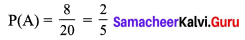Question 2.
One card is drawn from a pack of 52 cards, each of the 52 cards being equally likely to be drawn. Find the probability that the card drawn is
(i) an ace,
(ii) either red card or king.
Solution: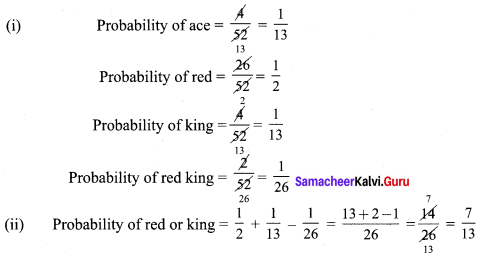Question 3.
A bag contains 3 red and 2 blue marbles. A marble is drawn at random. What is the probability of drawing a blue marble?
Solution: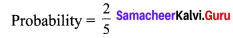Question 4.
Two dice are thrown simultaneously. Find the probability of getting
(i) an even number as the sum.
(ii) a total of at least 10
(iii) a doublet of even number.
Solution: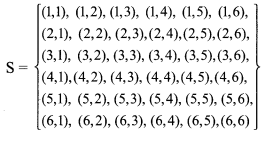n(S) = 36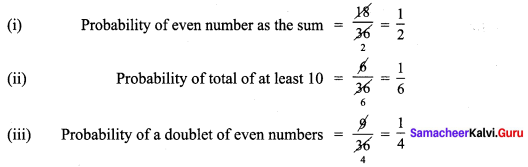Question 5.
An urn contains 10 red and 8 white balls. One ball is drawn at random. Find the probability that the ball drawn is white.
Solution: